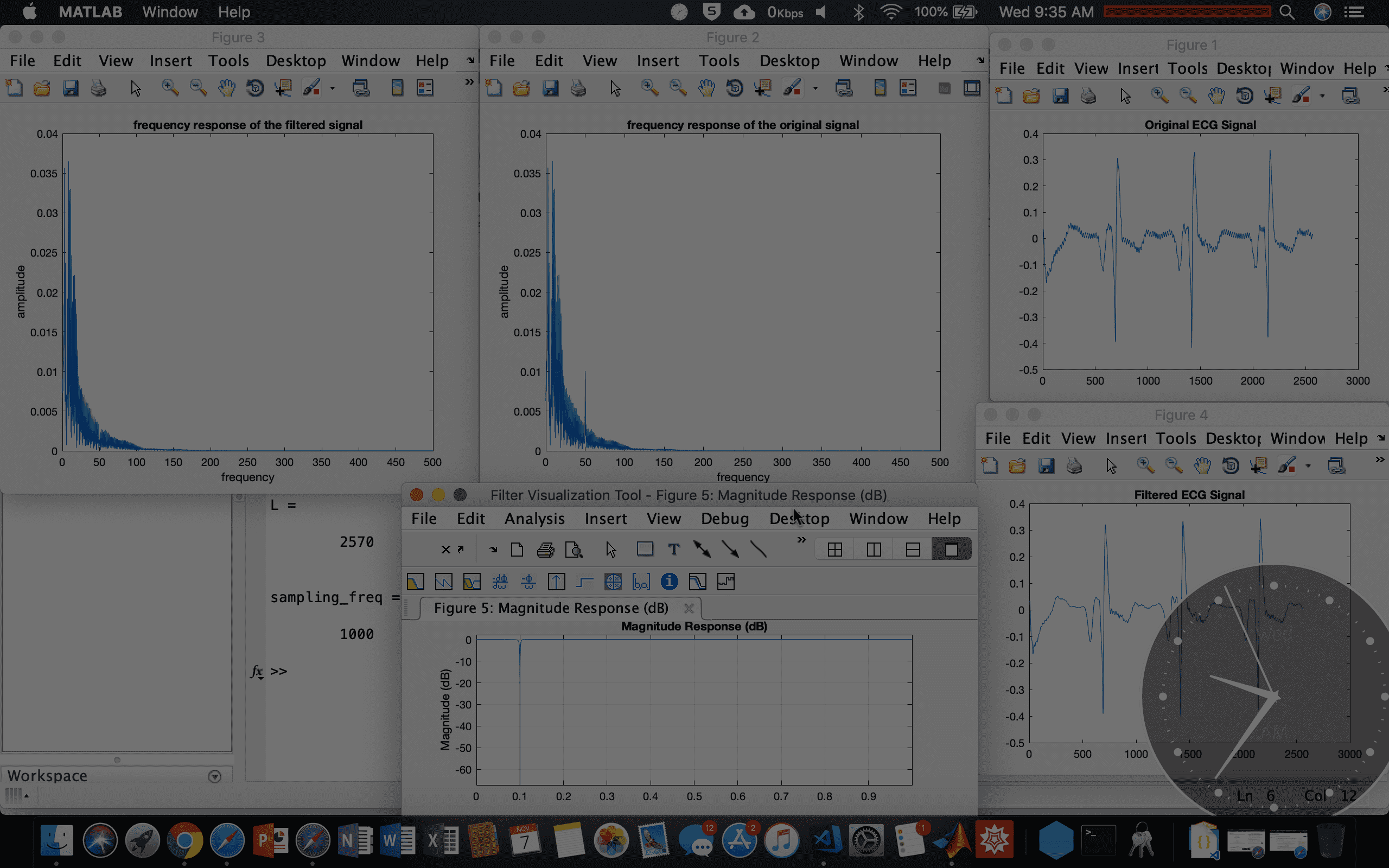Notch Filter to remove noise from an ECG Signal

# Notch Filter to remove noise from an ECG SignalThis experiment is based on a question at the Lab Exam (EN1093).

According to the following difference equation which relates output (y[n]) and input (x[n]) we were asked to derive the transfer function H(z). This filter removes the 50Hz noise component.

Pole-zero Plot